• 编译原理课后习题答案令A,B和C是任意正规式,证明以下关系成立(A|B)*=(A*B*)*=(A*|B*)*...
千次阅读
2019-10-06 03:49:46

## 题目：

令A、B和C是任意正规式，证明以下关系成立：

A∣A=A

(A*)*= A*

A*=ε∣A A*

(AB)*A=A(BA)*

(A∣B)*=(A*B*)*=(A*∣B*)*

A=b∣aA当且仅当A=a*b

### 解答：

（1）、A∣A=A

L(A∣A)=L(A)∪L(A)=L(A)，所以有A∣A=A。

（2）、(A*)*= A*

（3）、A*=ε∣A A*

通过证明两个正规式所表示的语言相同来证明两个正规式相等。

L(ε∣A A*)=L(ε)∪L(A)L(A*)= L(ε)∪L(A)(L(A) )*

=L(ε)∪L(A)((L(A))0∪(L(A))1∪(L(A))2∪(L(A))3∪…)

=L(ε)∪(L(A))1∪(L(A))2∪(L(A))3∪(L(A))4∪…

=(L(A))*=L(A*)

即：L(ε∣A A*)=L(A*)，所以有：A*=ε∣A A*

（4）、(AB)*A=A(BA)*

利用正规式的分配率和结合律直接推导。

(AB)*A=((AB)0∣(AB)1∣(AB)2∣(AB)3∣…)A

=εA∣(AB)1A∣(AB)2A∣(AB)3A∣…

=Aε∣A (BA)1∣A (BA)2∣A (BA)3∣…

=A(ε∣(BA)1∣(BA)2∣(BA)3∣…)

=A(BA)*

即：(AB)*A=A(BA)*

（5）、(A∣B)*=(A*B*)*=(A*∣B*)*

证明:先证(A∣B)*=(A*B*)*

因为L(A)L(A) *L(B) *,L(B)  L(A) *L(B) *

故:L(A) ∪L(B) L(A) *L(B) *

于是由本题第二小题结论可知(L(A)∪L(B)) *(L(A) *L(B)*)*      ①

又L(A)L(A)∪L(B),  L(B) L(A)∪L(B)

故:L(A)*(L(A)∪L(B))*

L(B)*(L(A)∪L(B))*

因此有:L(A)*L(B)* (L(A)∪L(B))* (L(A)∪L(B))*=( (L(A)∪L(B))*) 2

故(L(A)*L(B)*)*((L(A)∪L(B))*)*

由本题第二小题得: ((L(A)∪L(B))*)*= (L(A)∪L(B)) *

故得: (L(A)*L(B)*)*(L(A)∪L(B)) *             ②

则由①②得: (L(A)∪L(B)) *=(L(A)*L(B)*)*

由于L((A*B*))*=(L(A*B*))*=(L(A*)L(B*))*=(L(A)*L(B)*)*

即有(L(A)∪L(B))*=L((A*B*))*               ③

而(A|B)*对应的语言为(L(A)∪L(B))*,且(A*B*)*对应的语言为L((A*B*))*

则根据③得(A|B)*=(A*B*)*

再证:(A*|B*)*=(A*B*)*

因为:A,B是任意正规式,由以上结论得: (A*|B*)*=((A*)*(B*)*)*

又由本题第二小题目的结论可得：（A*）*=A*，（B*）*=B*

因此，（A*|B*）*=（A*B*）*

综合上述两种结论，最后得：(A∣B)*=(A*B*)*=(A*∣B*)*

转载于:https://www.cnblogs.com/hk-ming/p/5862743.html

更多相关内容
• ## 用Matlab实现A*算法和Dijkstra算法

万次阅读 多人点赞 2019-02-26 20:03:20
1. A*算法的伪代码 2. Dijkstra算法的伪代码 3. 具体实现 3.1 AStarGrid.m文件 function [route,numExpanded] = AStarGrid (input_map, start_coords, dest_coords) % Run A* algorithm on a grid. % ...

# 1. A*算法的伪代码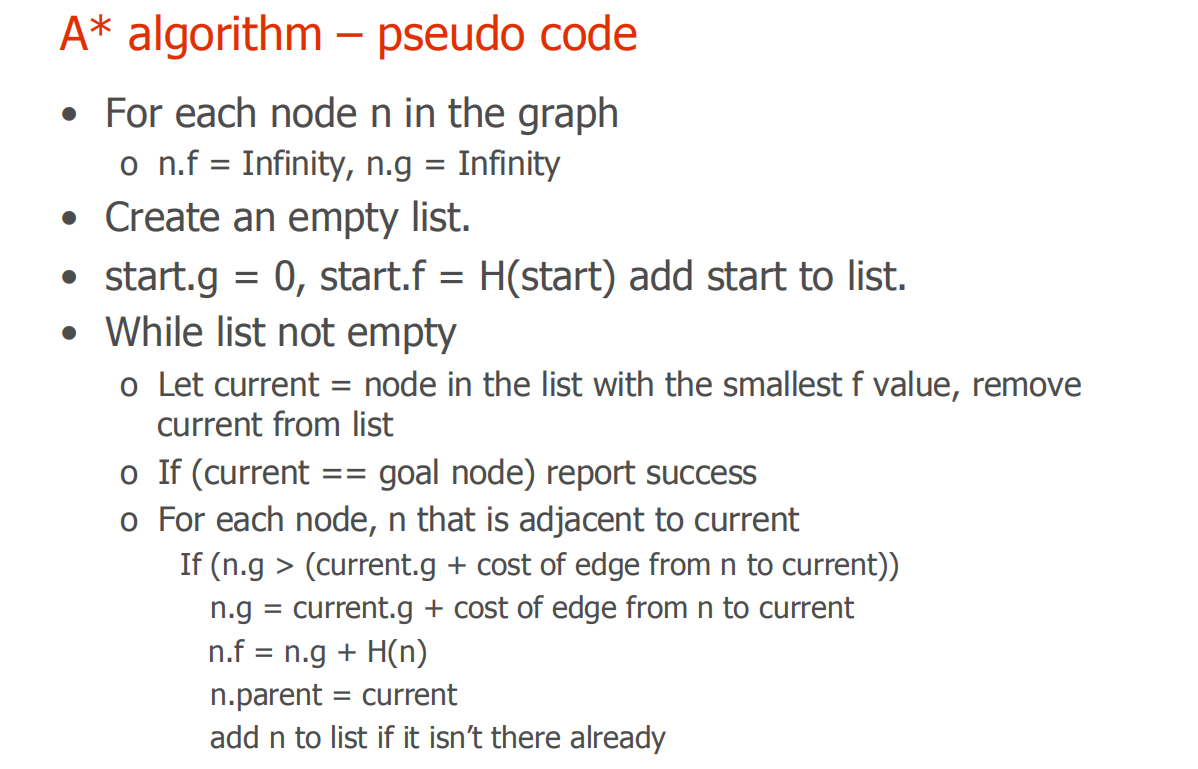# 2. Dijkstra算法的伪代码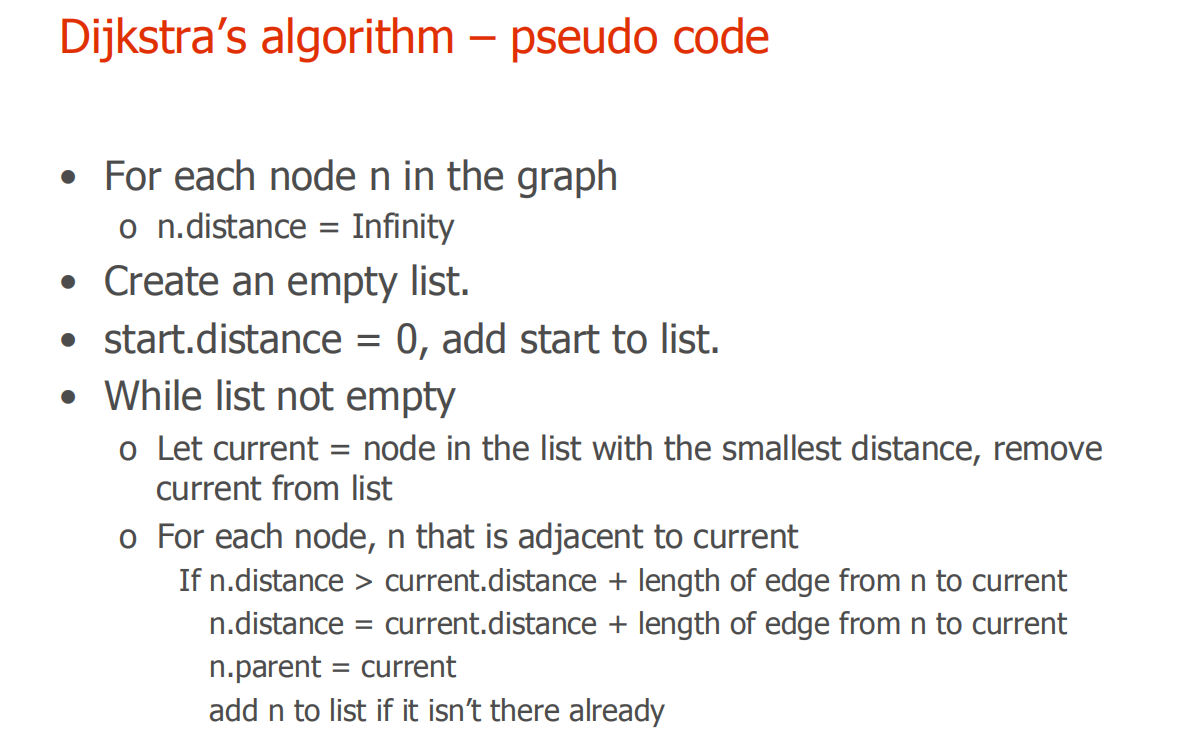# 3. 具体实现

## 3.1 AStarGrid.m文件

function [route,numExpanded] = AStarGrid (input_map, start_coords, dest_coords)
% Run A* algorithm on a grid.
% Inputs :
%   input_map : a logical array where the freespace cells are false or 0 and
%   the obstacles are true or 1
%   start_coords and dest_coords : Coordinates of the start and end cell
%   respectively, the first entry is the row and the second the column.
% Output :
%    route : An array containing the linear indices of the cells along the
%    shortest route from start to dest or an empty array if there is no
%    route. This is a single dimensional vector
%    numExpanded: Remember to also return the total number of nodes
%    expanded during your search. Do not count the goal node as an expanded node.

% set up color map for display用一个map矩阵来表示每个点的状态
% 1 - white - clear cell
% 2 - black - obstacle
% 3 - red = visited 相当于CLOSED列表的作用
% 4 - blue  - on list 相当于OPEN列表的作用
% 5 - green - start
% 6 - yellow - destination

cmap = [1 1 1; ...
0 0 0; ...
1 0 0; ...
0 0 1; ...
0 1 0; ...
1 1 0; ...
0.5 0.5 0.5];

colormap(cmap);

% variable to control if the map is being visualized on every
% iteration
drawMapEveryTime = true;

[nrows, ncols] = size(input_map);

% map - a table that keeps track of the state of each grid cell用来上色的
map = zeros(nrows,ncols);

map(~input_map) = 1;   % Mark free cells
map(input_map)  = 2;   % Mark obstacle cells

% Generate linear indices of start and dest nodes将下标转换为线性的索引值
start_node = sub2ind(size(map), start_coords(1), start_coords(2));
dest_node  = sub2ind(size(map), dest_coords(1),  dest_coords(2));

map(start_node) = 5;
map(dest_node)  = 6;

% meshgrid will replicate grid vectors' nrows and ncols to produce
% a full grid
% type help meshgrid' in the Matlab command prompt for more information
parent = zeros(nrows,ncols);%用来记录每个节点的父节点

%
[X, Y] = meshgrid (1:ncols, 1:nrows);

xd = dest_coords(1);
yd = dest_coords(2);

% Evaluate Heuristic function, H, for each grid cell
% Manhattan distance用曼哈顿距离作为启发式函数
H = abs(X - xd) + abs(Y - yd);
H = H';
% Initialize cost arrays
f = Inf(nrows,ncols);
g = Inf(nrows,ncols);

g(start_node) = 0;
f(start_node) = H(start_node);

% keep track of the number of nodes that are expanded
numExpanded = 0;

% Main Loop

while true

% Draw current map
map(start_node) = 5;
map(dest_node) = 6;

% make drawMapEveryTime = true if you want to see how the
% nodes are expanded on the grid.
if (drawMapEveryTime)
image(1.5, 1.5, map);
grid on;
axis image;
drawnow;
end

% Find the node with the minimum f value,其中的current是index值，需要转换
[min_f, current] = min(f(:));

if ((current == dest_node) || isinf(min_f))
break;
end;

% Update input_map
map(current) = 3;
f(current) = Inf; % remove this node from further consideration
numExpanded=numExpanded+1;
% Compute row, column coordinates of current node
[i, j] = ind2sub(size(f), current);

% *********************************************************************
% ALL YOUR CODE BETWEEN THESE LINES OF STARS
% Visit all of the neighbors around the current node and update the
% entries in the map, f, g and parent arrays
%
action=[-1 0; 1 0; 0 -1; 0 1];%上，下，左，右
for a=1:4
expand=[i,j]+action(a,:);
expand1=expand(1,1);
expand2=expand(1,2);
%不超出边界，不穿越障碍，不在CLOSED列表里，也不是起点，则进行扩展
if ( expand1>=1 && expand1<=10 && expand2>=1 && expand2<=10 && map(expand1,expand2)~=2 && map(expand1,expand2)~=3 && map(expand1,expand2)~=5)
if ( g(expand1,expand2)> g(i,j)+1 )
g(expand1,expand2)= g(i,j)+1;
f(expand1,expand2)= g(expand1,expand2)+H(expand1,expand2);
parent(expand1,expand2)=current;
map(expand1,expand2)=4;
end
end
end
%*********************************************************************

end

%% Construct route from start to dest by following the parent links
if (isinf(f(dest_node)))
route = [];
else
route = [dest_node];

while (parent(route(1)) ~= 0)
route = [parent(route(1)), route];
end

% Snippet of code used to visualize the map and the path
for k = 2:length(route) - 1
map(route(k)) = 7;
pause(0.1);
image(1.5, 1.5, map);
grid on;
axis image;
end
end

end



## 3.2 DijkstraGrid.m文件

function [route,numExpanded] = DijkstraGrid (input_map, start_coords, dest_coords)
% Run Dijkstra's algorithm on a grid.
% Inputs :
%   input_map : a logical array where the freespace cells are false or 0 and
%   the obstacles are true or 1
%   start_coords and dest_coords : Coordinates of the start and end cell
%   respectively, the first entry is the row and the second the column.
% Output :
%    route : An array containing the linear indices of the cells along the
%    shortest route from start to dest or an empty array if there is no
%    route. This is a single dimensional vector
%    numExpanded: Remember to also return the total number of nodes
%    expanded during your search. Do not count the goal node as an expanded node.

% set up color map for display
% 1 - white - clear cell
% 2 - black - obstacle
% 3 - red = visited
% 4 - blue  - on list
% 5 - green - start
% 6 - yellow - destination

cmap = [1 1 1; ...
0 0 0; ...
1 0 0; ...
0 0 1; ...
0 1 0; ...
1 1 0; ...
0.5 0.5 0.5];

colormap(cmap);

% variable to control if the map is being visualized on every
% iteration
drawMapEveryTime = true;

[nrows, ncols] = size(input_map);

% map - a table that keeps track of the state of each grid cell
map = zeros(nrows,ncols);

map(~input_map) = 1;   % Mark free cells
map(input_map)  = 2;   % Mark obstacle cells

% Generate linear indices of start and dest nodes
start_node = sub2ind(size(map), start_coords(1), start_coords(2));
dest_node  = sub2ind(size(map), dest_coords(1),  dest_coords(2));

map(start_node) = 5;
map(dest_node)  = 6;

% Initialize distance array
distanceFromStart = Inf(nrows,ncols);

% For each grid cell this array holds the index of its parent
parent = zeros(nrows,ncols);

distanceFromStart(start_node) = 0;

% keep track of number of nodes expanded
numExpanded = 0;

% Main Loop
while true

% Draw current map
map(start_node) = 5;
map(dest_node) = 6;

% make drawMapEveryTime = true if you want to see how the
% nodes are expanded on the grid.
if (drawMapEveryTime)
image(1.5, 1.5, map);
grid on;
axis image;
drawnow;
end

% Find the node with the minimum distance
[min_dist, current] = min(distanceFromStart(:));

if ((current == dest_node) || isinf(min_dist))
break;
end;

% Update map
map(current) = 3;         % mark current node as visited
numExpanded=numExpanded+1;
% Compute row, column coordinates of current node
[i, j] = ind2sub(size(distanceFromStart), current);

% *********************************************************************
% YOUR CODE BETWEEN THESE LINES OF STARS

% Visit each neighbor of the current node and update the map, distances
% and parent tables appropriately.
action=[-1 0; 1 0; 0 -1; 0 1];%上，下，左，右
for a=1:4
expand=[i,j]+action(a,:);
expand1=expand(1,1);
expand2=expand(1,2);
%不超出边界，不穿越障碍，不在CLOSED列表里，则进行扩展
if ( expand1>=1 && expand1<=10 && expand2>=1 && expand2<=10 && map(expand1,expand2)~=2 && map(expand1,expand2)~=3 && map(expand1,expand2)~=5 )
if ( distanceFromStart(expand1,expand2)> distanceFromStart(i,j)+1 )
distanceFromStart(expand1,expand2)= distanceFromStart(i,j)+1;
parent(expand1,expand2)=current;
map(expand1,expand2)=4;
end
end
end
distanceFromStart(current) = Inf; % remove this node from further consideration
%*********************************************************************

end

%% Construct route from start to dest by following the parent links
if (isinf(distanceFromStart(dest_node)))
route = [];
else
route = [dest_node];

while (parent(route(1)) ~= 0)
route = [parent(route(1)), route];
end

% Snippet of code used to visualize the map and the path
for k = 2:length(route) - 1
map(route(k)) = 7;
pause(0.1);
image(1.5, 1.5, map);
grid on;
axis image;
end
end

end



## 3.3 TestScript1.m文件

%
% TestScript for Assignment 1
%

%% Define a small map
map = false(10);

map (1:5, 6) = true;

start_coords = [6, 2];
dest_coords  = [8, 9];

%%
close all;
[route, numExpanded] = DijkstraGrid (map, start_coords, dest_coords);
% Uncomment following line to run Astar
% [route, numExpanded] = AStarGrid (map, start_coords, dest_coords);

%HINT: With default start and destination coordinates defined above, numExpanded for Dijkstras should be 76, numExpanded for Astar should be 23.



# 4. 路径显示

运行TestScript1，可得如下路径（绿色代表起点，黄色代表终点，黑色代表障碍，红色代表CLOSED列表，蓝色代表OPEN列表）：

## 4.1 A*算法## 4.2 Dijkstra算法展开全文Matlab Dijkstra 路径规划
• 先了解一下什么是A*算法。 A*搜寻算法，俗称A星算法。这是一种在图形平面上，有多个节点的路径，求出最低通过成本的算法。常用于游戏中的NPC（Non-Player-ControlledCharacter）的移动计算，或线上游戏的BOT...

先了解一下什么是A*算法。

A*搜寻算法，俗称A星算法。这是一种在图形平面上，有多个节点的路径，求出最低通过成本的算法。常用于游戏中的NPC（Non-Player-ControlledCharacter）的移动计算，或线上游戏的BOT（ROBOT）的移动计算上。该算法像Dijkstra算法一样，可以找到一条最短路径；也像BFS一样，进行启发式的搜索。
A*算法是一种启发式搜索算法，启发式搜索就是在状态空间中的搜索对每一个搜索的位置进行评估，得到最好的位置，再从这个位置进行搜索直到目标。这样可以省略大量无谓的搜索路径，提高了效率。

## A星算法核心公式：

F = G + H

F - 方块的总移动代价
G - 开始点到当前方块的移动代价
H - 当前方块到结束点的预估移动代价

G值是怎么计算的？
假设现在我们在某一格子，邻近有8个格子可走，当我们往上、下、左、右这4个格子走时，移动代价为10；当往左上、左下、右上、右下这4个格子走时，移动代价为14；即走斜线的移动代价为走直线的1.4倍。
这就是G值最基本的计算方式，适用于大多数2.5Drpg页游。
根据游戏需要，G值的计算可以进行拓展。如加上地形因素对寻路的影响。格子地形不同，那么选择通过不同地形格子，移动代价肯定不同。同一段路，平地地形和丘陵地形，虽然都可以走，但平地地形显然更易走。
我们可以给不同地形赋予不同代价因子，来体现出G值的差异。如给平地地形设置代价因子为1，丘陵地形为2，在移动代价相同情况下，平地地形的G值更低，算法就会倾向选择G值更小的平地地形。

## 拓展公式：

G = 移动代价 * 代价因子

H值是如何预估出来的？
很显然，在只知道当前点，结束点，不知道这两者的路径情况下，我们无法精确地确定H值大小，所以只能进行预估。
有多种方式可以预估H值，如曼哈顿距离、欧式距离、对角线估价，最常用最简单的方法就是使用曼哈顿距离进行预估：
H = 当前方块到结束点的水平距离 + 当前方块到结束点的垂直距离
题外话：A星算法之所以被认为是具有启发策略的算法，在于其可通过预估H值，降低走弯路的可能性，更容易找到一条更短的路径。其他不具有启发策略的算法，没有做预估处理，只是穷举出所有可通行路径，然后从中挑选一条最短的路径。这也是A星算法效率更高的原因。

鉴于前人已经把原理讲的很清楚了，便不再废话，想要深入了解下的可以参考下面的两篇文章。

接下来上代码：

## 代码1

文件AStar.py

# coding=utf-8

#描述AStar算法中的节点数据
class Point:
"""docstring for point"""
def __init__(self, x = 0, y = 0):
self.x = x
self.y = y

class Node:
def __init__(self, point, g = 0, h = 0):
self.point = point        #自己的坐标
self.father = None        #父节点
self.g = g                #g值
self.h = h                #h值

"""
估价公式：曼哈顿算法
"""
def manhattan(self, endNode):
self.h = (abs(endNode.point.x - self.point.x) + abs(endNode.point.y - self.point.y))*10

def setG(self, g):
self.g = g

def setFather(self, node):
self.father = node

class AStar:
"""
A* 算法
python 2.7
"""
def __init__(self, map2d, startNode, endNode):
"""
map2d:      寻路数组
startNode:  寻路起点
endNode:    寻路终点
"""
#开放列表
self.openList = []
#封闭列表
self.closeList = []
#地图数据
self.map2d = map2d
#起点
self.startNode = startNode
#终点
self.endNode = endNode
#当前处理的节点
self.currentNode = startNode
#最后生成的路径
self.pathlist = [];
return;

def getMinFNode(self):
"""
获得openlist中F值最小的节点
"""
nodeTemp = self.openList
for node in self.openList:
if node.g + node.h < nodeTemp.g + nodeTemp.h:
nodeTemp = node
return nodeTemp

def nodeInOpenlist(self,node):
for nodeTmp in self.openList:
if nodeTmp.point.x == node.point.x \
and nodeTmp.point.y == node.point.y:
return True
return False

def nodeInCloselist(self,node):
for nodeTmp in self.closeList:
if nodeTmp.point.x == node.point.x \
and nodeTmp.point.y == node.point.y:
return True
return False

def endNodeInOpenList(self):
for nodeTmp in self.openList:
if nodeTmp.point.x == self.endNode.point.x \
and nodeTmp.point.y == self.endNode.point.y:
return True
return False

def getNodeFromOpenList(self,node):
for nodeTmp in self.openList:
if nodeTmp.point.x == node.point.x \
and nodeTmp.point.y == node.point.y:
return nodeTmp
return None

def searchOneNode(self,node):
"""
搜索一个节点
x为是行坐标
y为是列坐标
"""
#忽略障碍
if self.map2d.isPass(node.point) != True:
return
#忽略封闭列表
if self.nodeInCloselist(node):
return
#G值计算
if abs(node.point.x - self.currentNode.point.x) == 1 and abs(node.point.y - self.currentNode.point.y) == 1:
gTemp = 14
else:
gTemp = 10

#如果不再openList中，就加入openlist
if self.nodeInOpenlist(node) == False:
node.setG(gTemp)
#H值计算
node.manhattan(self.endNode);
self.openList.append(node)
node.father = self.currentNode
#如果在openList中，判断currentNode到当前点的G是否更小
#如果更小，就重新计算g值，并且改变father
else:
nodeTmp = self.getNodeFromOpenList(node)
if self.currentNode.g + gTemp < nodeTmp.g:
nodeTmp.g = self.currentNode.g + gTemp
nodeTmp.father = self.currentNode
return;

def searchNear(self):
"""
搜索节点周围的点
按照八个方位搜索
拐角处无法直接到达
(x-1,y-1)(x-1,y)(x-1,y+1)
(x  ,y-1)(x  ,y)(x  ,y+1)
(x+1,y-1)(x+1,y)(x+1,y+1)
"""
if self.map2d.isPass(Point(self.currentNode.point.x - 1, self.currentNode.point.y)) and \
self.map2d.isPass(Point(self.currentNode.point.x, self.currentNode.point.y -1)):
self.searchOneNode(Node(Point(self.currentNode.point.x - 1, self.currentNode.point.y - 1)))

self.searchOneNode(Node(Point(self.currentNode.point.x - 1, self.currentNode.point.y)))

if self.map2d.isPass(Point(self.currentNode.point.x - 1, self.currentNode.point.y)) and \
self.map2d.isPass(Point(self.currentNode.point.x, self.currentNode.point.y + 1)):
self.searchOneNode(Node(Point(self.currentNode.point.x - 1, self.currentNode.point.y + 1)))

self.searchOneNode(Node(Point(self.currentNode.point.x, self.currentNode.point.y - 1)))
self.searchOneNode(Node(Point(self.currentNode.point.x, self.currentNode.point.y + 1)))

if self.map2d.isPass(Point(self.currentNode.point.x, self.currentNode.point.y - 1)) and \
self.map2d.isPass(Point(self.currentNode.point.x + 1, self.currentNode.point.y)):
self.searchOneNode(Node(Point(self.currentNode.point.x + 1, self.currentNode.point.y - 1)))

self.searchOneNode(Node(Point(self.currentNode.point.x + 1, self.currentNode.point.y)))

if self.map2d.isPass(Point(self.currentNode.point.x + 1, self.currentNode.point.y)) and \
self.map2d.isPass(Point(self.currentNode.point.x, self.currentNode.point.y + 1)):
self.searchOneNode(Node(Point(self.currentNode.point.x + 1, self.currentNode.point.y + 1)))
return;

def start(self):
'''''
开始寻路
'''
#将初始节点加入开放列表
self.startNode.manhattan(self.endNode);
self.startNode.setG(0);
self.openList.append(self.startNode)

while True:
#获取当前开放列表里F值最小的节点
#并把它添加到封闭列表，从开发列表删除它
self.currentNode = self.getMinFNode()
self.closeList.append(self.currentNode)
self.openList.remove(self.currentNode)

self.searchNear();

#检验是否结束
if self.endNodeInOpenList():
nodeTmp = self.getNodeFromOpenList(self.endNode)
while True:
self.pathlist.append(nodeTmp);
if nodeTmp.father != None:
nodeTmp = nodeTmp.father
else:
return True;
elif len(self.openList) == 0:
return False;
return True;

def setMap(self):
for node in self.pathlist:
self.map2d.setMap(node.point);
return;

## 文件2

文件map2d.py

# coding=utf-8
from __future__ import print_function

class map2d:
"""
地图数据
"""
def __init__(self):
self.data = [list("####################"),
list("#*****#************#"),
list("#*****#*****#*####*#"),
list("#*########*##******#"),
list("#*****#*****######*#"),
list("#*****#####*#******#"),
list("####**#*****#*######"),
list("#*****#**#**#**#***#"),
list("#**#*****#**#****#*#"),
list("####################")]

self.w = 20
self.h = 10
self.passTag = '*'
self.pathTag = 'o'

def showMap(self):
for x in xrange(0, self.h):
for y in xrange(0, self.w):
print(self.data[x][y], end='')
print(" ")
return;

def setMap(self, point):
self.data[point.x][point.y] = self.pathTag
return;

def isPass(self, point):
if (point.x < 0 or point.x > self.h - 1) or (point.y < 0 or point.y > self.w - 1):
return False;

if self.data[point.x][point.y] == self.passTag:
return True;

## 文件3

文件AStarTest.py

# coding=utf-8
import map2d
import AStar

if __name__ == '__main__':
##构建地图
mapTest = map2d.map2d();
mapTest.showMap();
##构建A*
aStar = AStar.AStar(mapTest, AStar.Node(AStar.Point(1,1)), AStar.Node(AStar.Point(8,18)))
print "A* start:"
##开始寻路
if aStar.start():
aStar.setMap();
mapTest.showMap();
else:
print "no way"

在AStar.py中增加了对拐角的处理，设置拐角无法直达。

## 运行结果：参考：

# A星算法详解(个人认为最详细,最通俗易懂的一个版本)

展开全文深度学习 python
• ## A算法和A*算法详解

万次阅读 多人点赞 2019-12-02 18:07:08
A算法和A*算法都适用 1、用初始节点初始化搜索图G （动态变化），将初始节点放入open表（还没有扩展的节点）中，然后初试closed（已经扩展完成的节点）表赋空NULL 2、如果open表不为空进入循环 2.1 将open表中的第...

字太多了 直接放笔记的图片吧，如有不对请指正

A算法和A*算法都适用
1、用初始节点初始化搜索图G （动态变化），将初始节点放入open表（还没有扩展的节点）中，然后初试closed（已经扩展完成的节点）表赋空NULL
2、如果open表不为空进入循环
2.1 将open表中的第一个元素的指针赋给临时变量n表示当前遍历的节点，然后将当前节点n的指针假如到closed表表示扩展完成
2.2 如果当前节点n是目标节点，任务完成 结束算法
2.3 扩展当前节点n
使用临时集合M存储n后面的若干个（≥1）子节点m，且m不可以是n的祖先
将解图G并上M，表示前一轮循环定义的解空间+当前节点所能得到的可能的后续节点
2.4 判断M中的节点m，
如果m不属于closed也不属于open则建立m——>n的指针，并将m节点加入到open表中
如果m属于open，则考虑是否修改m的指针，看n节点下m的f（n）和open表中m节点f（n）的大小
如果m属于closed，则考虑是否修改m以及G中后裔的指针
2.5 重新排列open表中的节点（根据估价函数）
下面是例子

图上面边和点的值在最后一张图有解释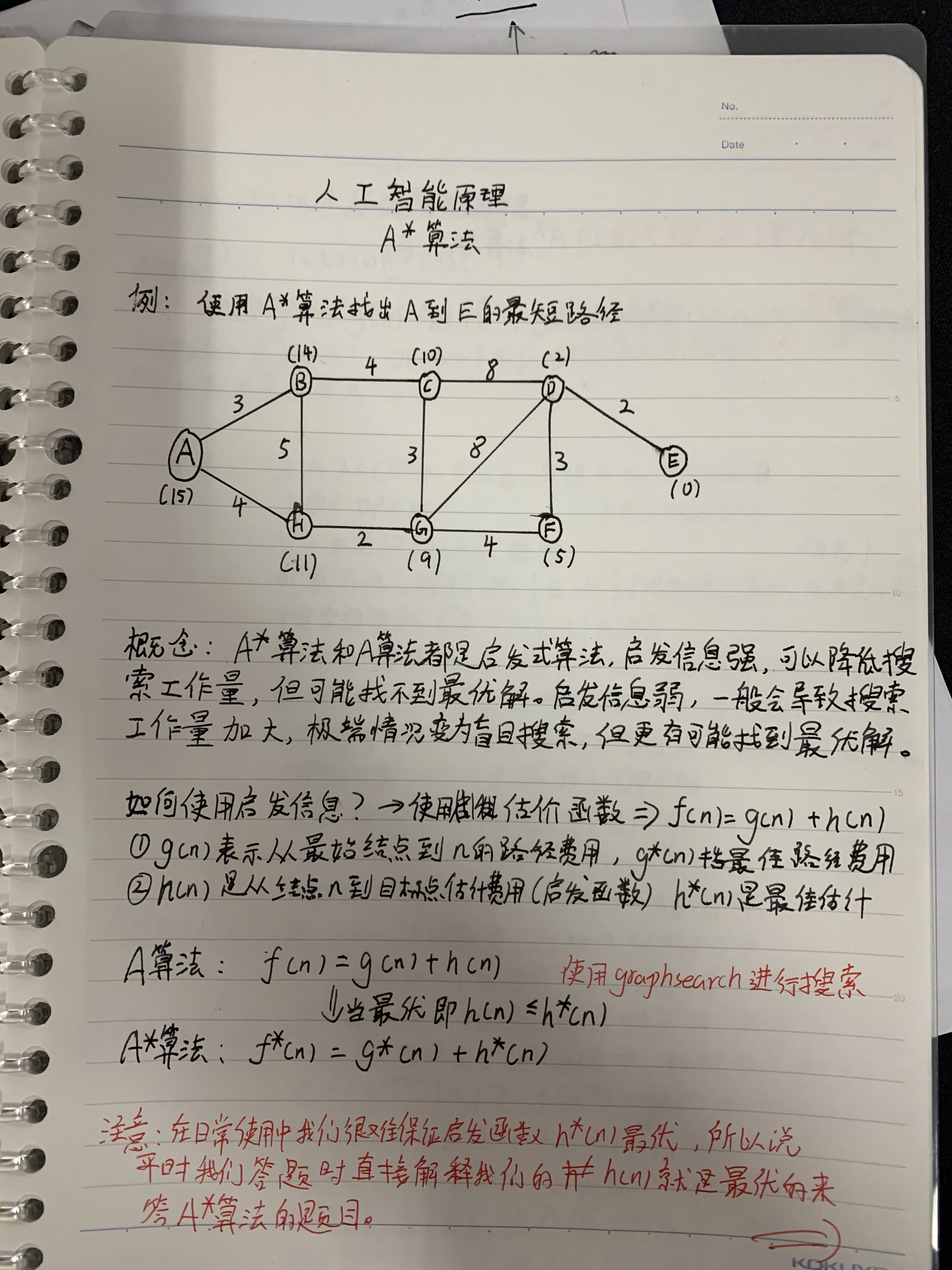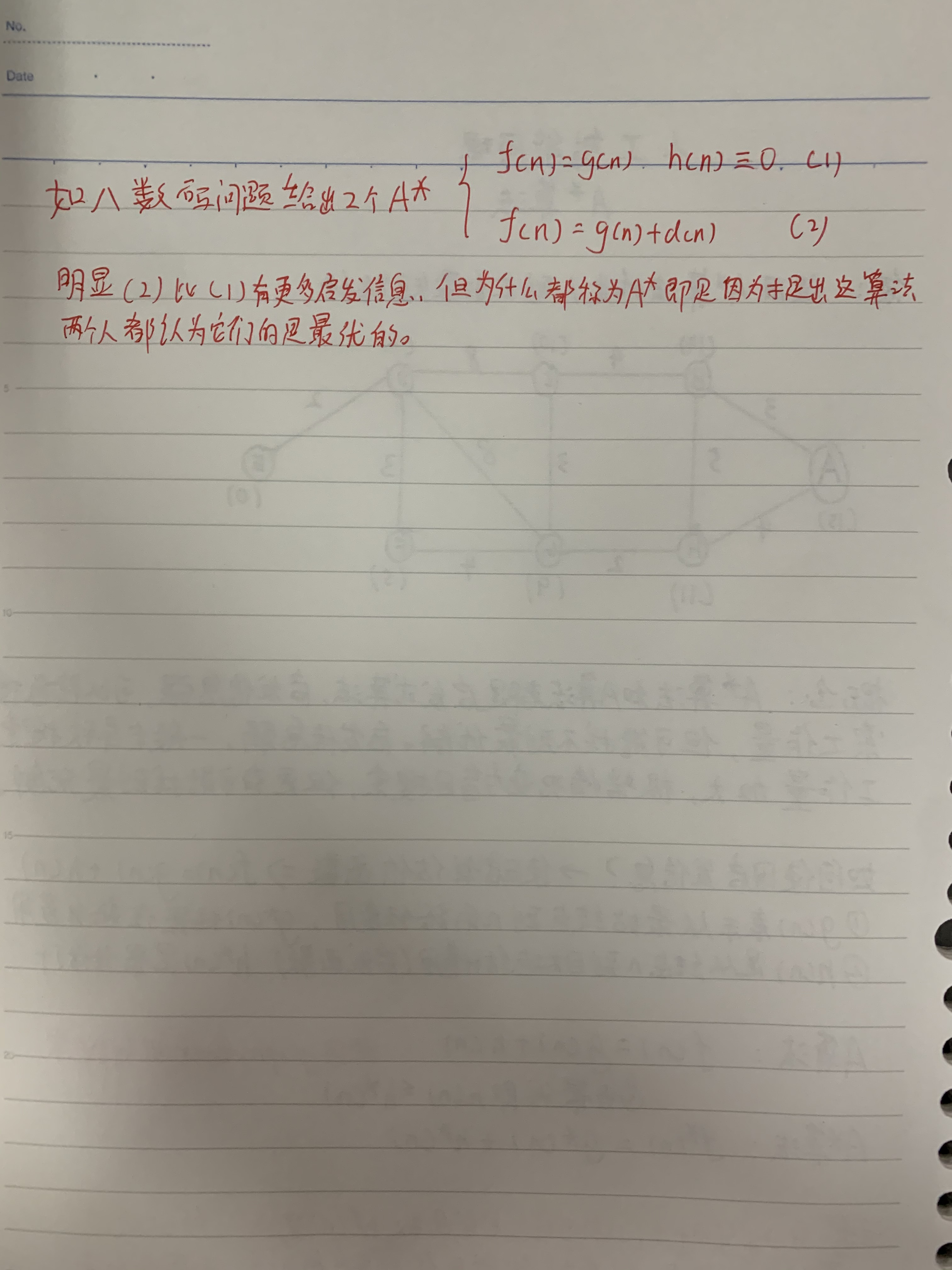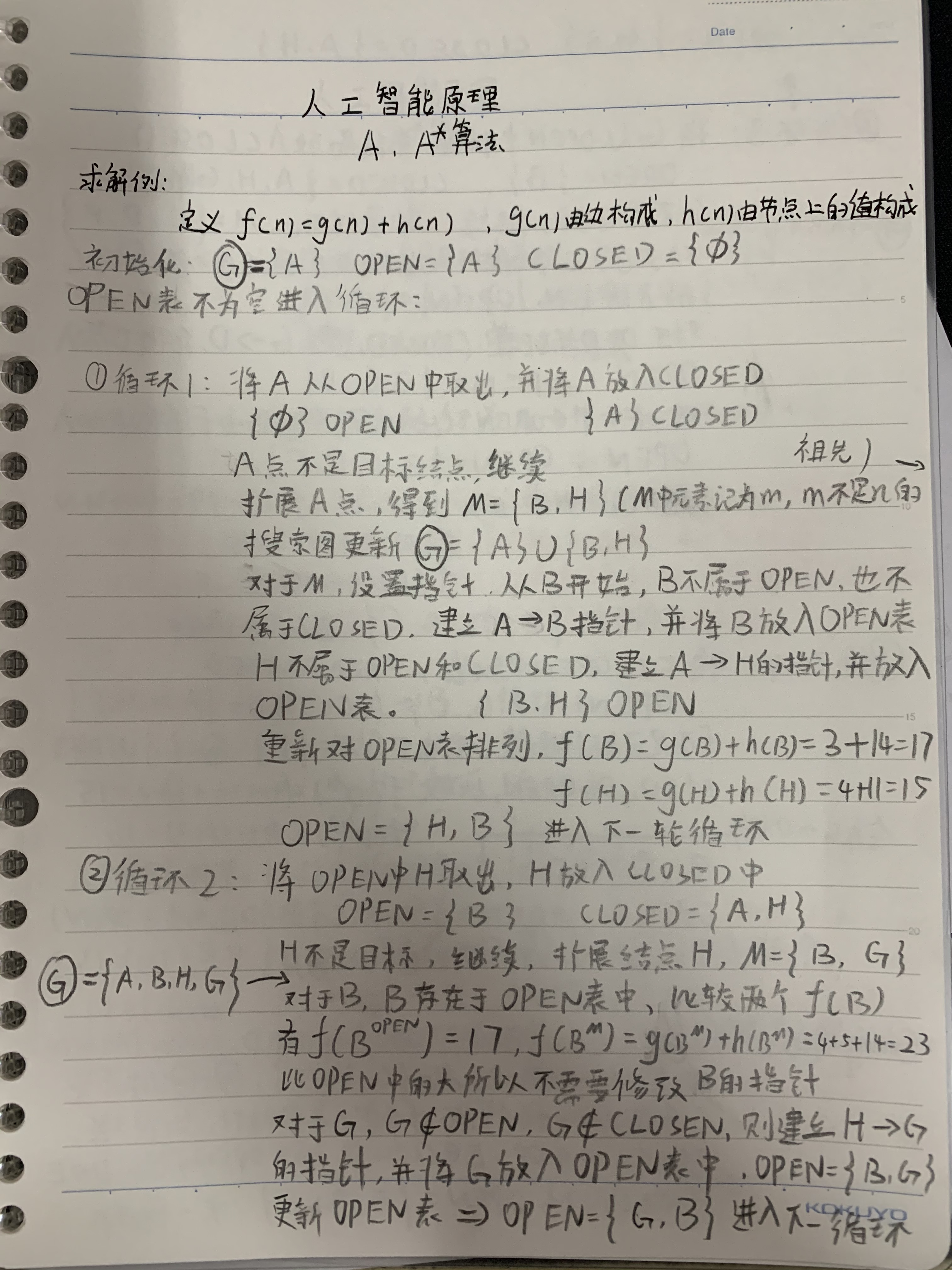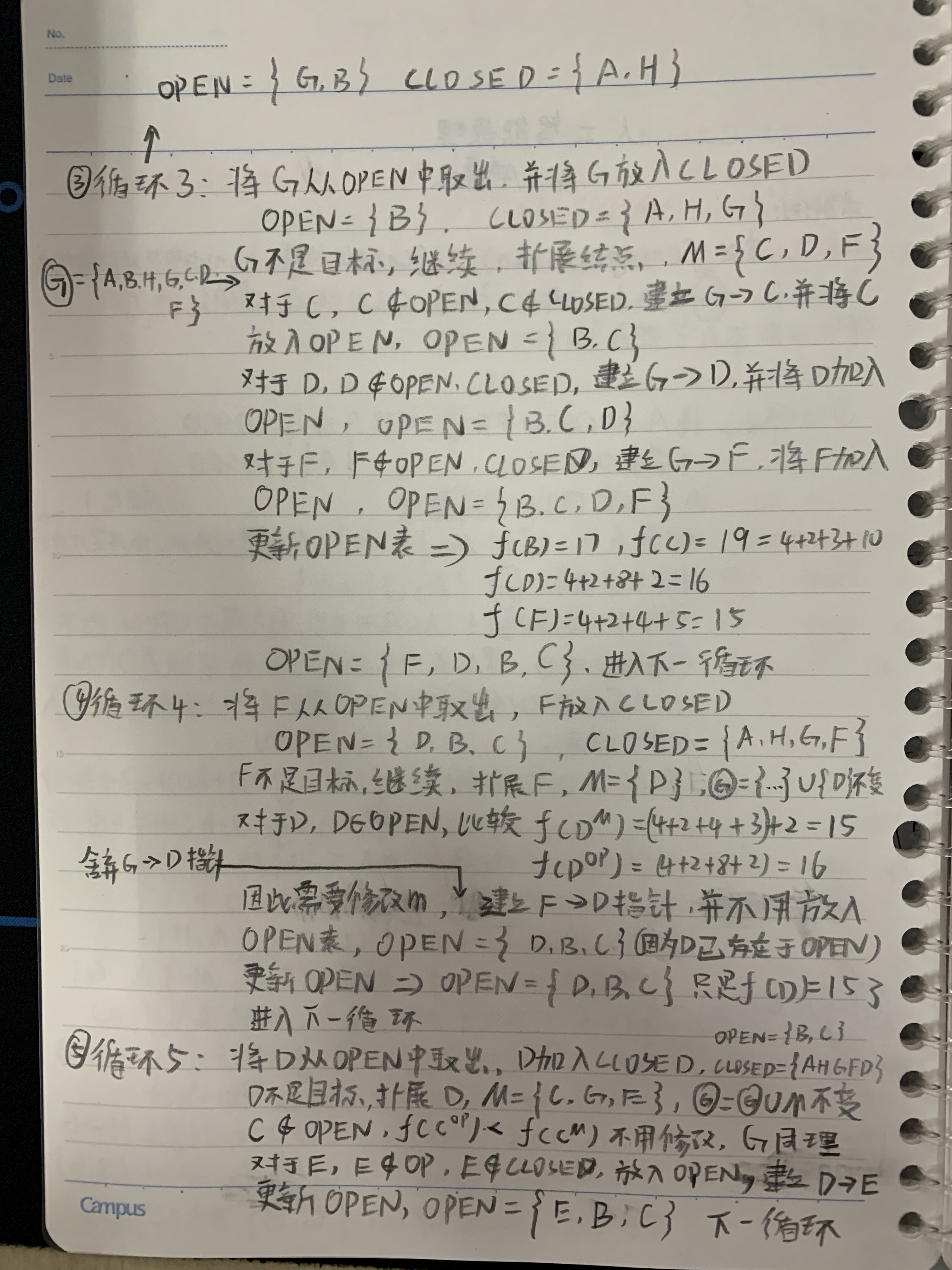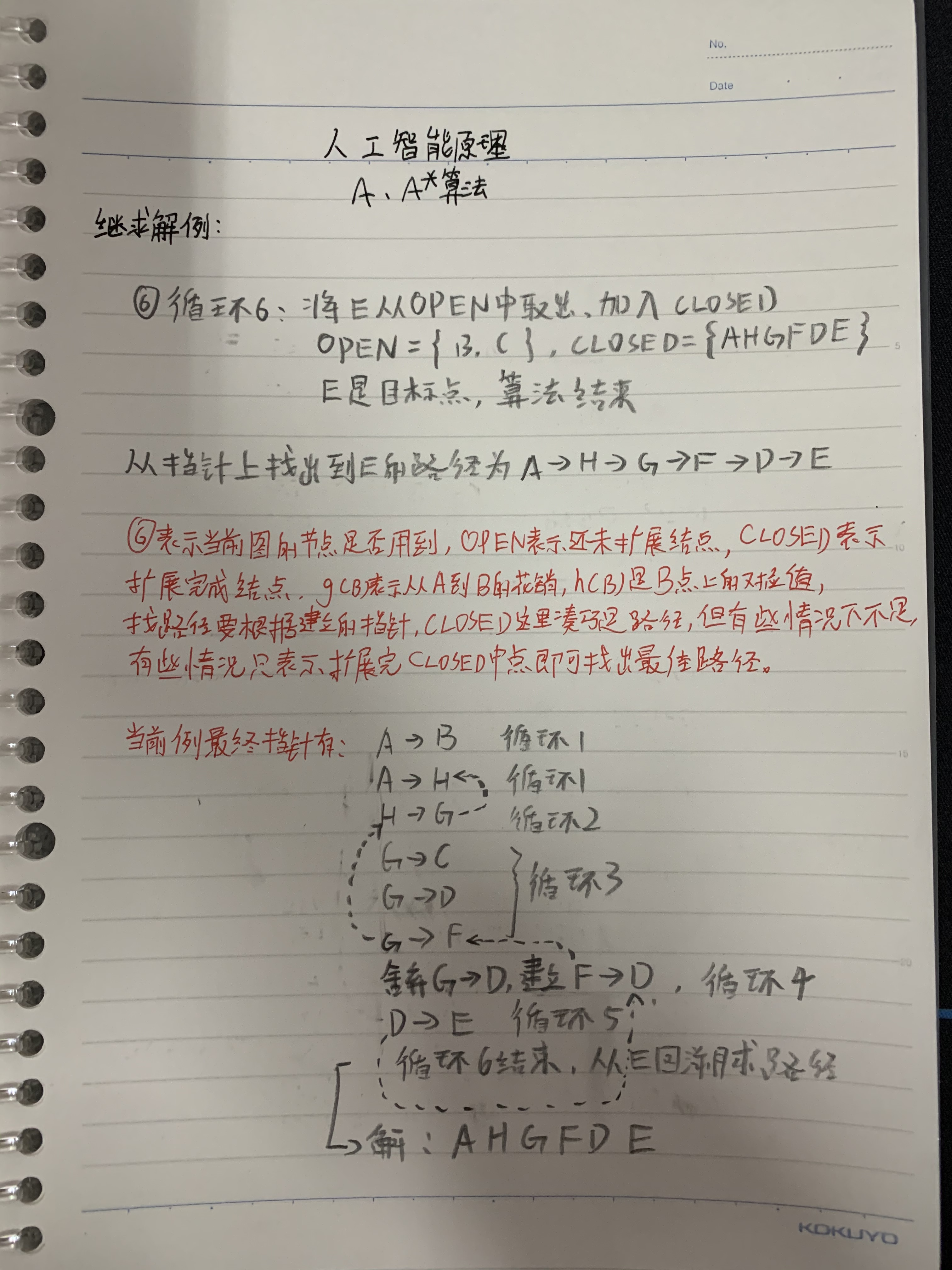展开全文• ## 路径规划A*算法

万次阅读 2017-12-10 11:42:17
A*算法是在Dijkstra算法上进行改进，毕竟，我们是知道终点和起点的位置信息的，Dijkstra算法完全是四面八方全都找，然而我们既然已经知道，比方说重点在起点的北方，那么完全可以直接往北方搜索。所以算法综合了Best...算法 搜索
• ## a += a-= a*a

万次阅读 多人点赞 2017-10-18 17:27:26
a += a-= a*a; 最终a的值是多少？ 这要分语言了。 1）在c语言中，结果是-12。原因是先算a*a（结果为9，此时a的值没变，还是3）；然后算 a-=a*a，等效于 a = a - 9;（结果为-6，因为赋值符号，此时a的值为-6，...C语言 java
• 这里用到一个条件：启发式函数h(s)所估计的s点到终点的距离，一定要小于等于实际中s点到终点的距离才行（不然第6步就不成立）。路径规划
• 目前ROS中可以使用的global planner主要包括：Dijkstra，A*和D*算法。local planner主要有：dwa、trajectory、teb和eband等。目前、teb local planner效果可能会好点。 一、Dijkstra算法 ...
• ## A*算法 matlab版代码

千次阅读 2018-11-21 10:40:07
A*算法用于路径规划，随机生成障碍、起点和终点，寻找最优路径 A* 算法是一种最优解算法，即如果起始点到目标点的最优路径存在，A* 算法能够保证找到该最优路径；但其所规划路径拐点多、拐角大，不符合机器人的运动...matlab
• ## C++(25)——A*B问题

千次阅读 2020-10-08 16:14:31
输入两个正整数A和B，求A*B。 输入 一行，包含两个正整数A和B，中间用单个空格隔开。1 <= A,B <= 50000。 输出 一个整数，即A*B的值。 样例输入 3 4 样例输出 12 提示 注意乘积的范围和数据类型的...
• ## hybrid a*(混合A星算法-hybrid a star)

万次阅读 多人点赞 2019-03-21 11:05:19
版权声明：本文为博主原创文章，转载注明来源。 2010年，斯坦福首次提出一种满足车辆...2、Hybrid A*A*区别 Hybrid A* A* 维数 H...
• ## A*算法的实现(Python)

千次阅读 热门讨论 2020-06-28 21:35:44
关于A*算法的实现是很早之前的一次开发中的成果，并做了一些改进。当然，在这里就不记录改进部分了，因为其中还有一些争议。这里仅是对A*算法的理解和使用Python实现。 定义 (百度百科)A*（A-Star)算法是一种...
• ## Matlab中的A.*B与A*B

千次阅读 2019-03-16 10:18:10
在进行矩阵乘法运算时，Matlab为我么提供...此运算与线性代数里的矩阵相乘计算方法一样，不需要A、B形状一样，但要满足A的列数与B的行数一样（如：A为mn矩阵，B为n*p矩阵）。 下面用实例来说明matlab中这两种乘法C=A...matlab语法
• A*算法是对A算法的估价函数 f(n)=g(n)+h(n) 加上某些限制后得到的一种启发式搜索算法 假设f*(n)是从初始结点S0出发，约束经过结点n到达目标结点Sg的最小代价，估价函数f(n)是对f*(n)的估计值。记 f*(n)=g*(n)+h*(n)...
• ## A*算法和dijkstra算法

万次阅读 2018-08-10 16:42:55
A*算法和dijkstra算法都是启发式搜索，dijkstra算法可以看成是广度优先搜索，而A*可以认为是深度优先搜索。 A*可以轻松地用在比如无人机航路规划中，而dijkstra建立在较为抽象的图论层面。 A*算法主要是有两张表，...
• A*、LPA*以及D* lite都可以用于静态环境下移动机器人的路径规划，此时三者计算效率都相差不大，都利用了启发式搜索来提高效率，LPA*和D* Lite的增量式搜索在这时没有任何帮助，但对于动态环境的路径规划，A*算法却...
• 今天用A*算法跑了一张1080*1920的地图，发现程序直接卡死了。 网上查到的结果做一总结 从00到1000复杂地形，竟然需要数分钟。精度越高，越费时间。 1000*1000的地图 首先得到开放表的8个点运算10次 判断此8个点...
• ## A算法与A*算法区别

万次阅读 多人点赞 2017-03-15 19:15:58算法
• ## 双向A*算法浅析

千次阅读 热门讨论 2017-08-22 22:17:19
言： 本文基于我写的A*浅析... 建议先看完A*浅析再看本文。 引入： 众所周知，双向BFS是对BFS极大的优化，它从起点和终点开始分别搜索，直到相遇。 那么，既然有双向BFS，为什么不能有双向A*呢？搜索优化
• ## Dijkstra算法和A*算法总结

万次阅读 多人点赞 2018-01-03 13:48:27
Dijkstra算法和A*算法都是最短路径问题的常用算法，下面就对这两种算法的特点进行一下比较。 1.Dijkstra算法计算源点到其他所有点的最短路径长度，A*关注点到点的最短路径(包括具体路径)。 2.Dijkstra算法建立在较为...dijkstra
• 摘要：本文针对机器人路径规划提出了两种算法，分析了基于栅格地图的Dijkstra算法...关键词：栅格地图、Dijkstra、A* 一. 引言 路径规划算法的研究是移动机器人研究领域中一个重要的组成部分，它的目的是使移动机器...
• ## 改进A*算法

千次阅读 2018-06-22 11:15:19
最短路径搜索问题是智能交通技术应用中的一个关键问题,而A*算法是一种静态路网中求解最短路径最有效的直接搜索方法.传统的A*算法未考虑到实际路网中交通灯的影响,求得的最短路径并不一定是行程时间最短.但是路径选取...
• ## A*算法中的估价函数

万次阅读 多人点赞 2017-11-15 21:41:24
这两天在编写人工智能大作业，主要是A*寻路算法这方面，加之考试中会涉及到估价函数这方面的考点，所以我就对A*算法中的估价函数做一下总结。 首先，先说下启发式搜索。在空间很大的情况下，如果只是采取广度优先...人工智能
• ## 多目标的A*算法路径规划

千次阅读 热门讨论 2019-04-13 20:37:44
• 没有必要刻意忙碌，那样心就不会思考了 疑惑 **2 **0.5是什么意思 解惑 注意了，两个*后面的数字是指数 **2 是求平方 **3是立方 **0.5是开根号 ...
• 最近要实现汽车实现简单的倒车入库，所以通过修改move base的调度机制，实现此功能。 第一步 第二步 第三步 流程图：规划调度机制 修改的源码的流程图 相关视频 ... ...
• 在同一行依次输入三个值a,b,c，用空格分开，输出 bb-4a*c的值 在同一行依次输入三个值a,b,c，用空格分开，输出 bb-4a*c的值 输入格式: 在一行中输入三个数。 输出格式: 在一行中输出公式值。...print(b*b-4*a*c) ...python
• ## A*寻路算法的最简单通俗讲解

万次阅读 多人点赞 2016-01-23 13:54:36
为了节约点眼泪，今天我们就来介绍著名的A*寻路算法（或简称A*算法）。 Peter Hart, Nils Nilsson 和 Bertram Raphael 在1968年首次提出了著名的A*算法。A*算法其实是Dijkstra最短路径算法的一种扩展。不一样的是...算法 最短路径
• 看到了hann(5)*hann(5)’ 这个操作却不知道是干啥的,一番实验之后才发现这个 ‘玩意是转置的意思。尴尬了。...